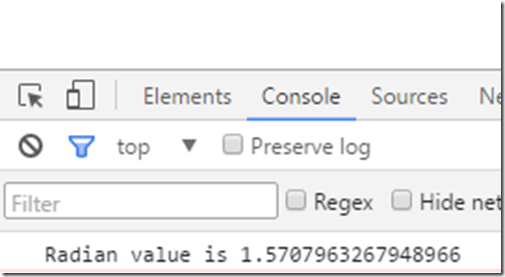# How to convert from Degree to Radian in JavaScript ?

Assume that you have an angle in degree and you wish to convert it to radians in JavaScript so that this can be used in some mathematical formulas.

# How to convert between Degrees and Radians in JavaScript ?

Just multiply the degree with the following formula to get the radian.

```<script type="text/javascript">
var degree = 90;
var radianOutput = degree * (Math.PI / 180);